A Genetic Algorithm Demo project

I first learned about Genetic Algorithms (GAs) in May 1989 when I read Simulated Evolution: wherein bugs learn to hunt bacteria by A. K. Dewdney in Scientific American. I wrote a program similar to the one described. I watched dots change their behavior. I was hooked. Since then I've written a large number of GAs, mostly just to see them work.

## Introduction

### What is a Genetic Algorithm?

I will give only the briefest answer to the question "What is a Genetic Algorithm?" since there are many fine sources of information available, many online. Wikipedia, for example. Those familiar with GAs may want to skip to the end.

A genetic algorithm is a biologically inspired trial-and-error search technique for qualifying potential solutions to a problem. It tries many solutions simultaneously, it is iterative, and it probabilistically favors the best solutions from the current iteration to seed the next.

### What methods are available for finding solutions?

If a problem can be described with one or more expressions in some number of variables, and if there is a method for evaluating the expressions for different values of the variables, then a search for an optimum solution can be performed in several different ways:
• Calculus based search
If the expressions are tractable to contemporary mathematical analysis, use a calculus based method and turn the proverbial crank.
• Since mathematicians are devices for turning caffeine into theorems, the large problems may require an espresso machine as part of the solution.
• An idiot savant on call wouldn't hurt.
• Enumerative search
If it is possible to exhaustively search all possible outcomes then this may be the preferred solution.
• Since computer programmers are devices for turning caffeine into programs, the large problems may require an espresso machine as part of the solution. Put it next to the Cray.
• An idiot savant on call wouldn't hurt.
• Random searches
Random walks and other random based search techniques. More later.

• Genetic algorithm
More on this later.

• Wait till later
Genetic algorithms would not have been on this list prior to 1975 when John Holland published Adaptation in Natural and Artificial Systems. So there may be something else available next year, and the solution can wait.

• "Intuitive" (a.k.a. No Solution)
Throw up your hands in the face of complexity and make a "gut feeling" decision.
• An MBA on call wouldn't hurt in this case.

When it is unacceptable to have no solution, when enumeration and mathematical analysis are not practical, and when a purely random technique would leave too many questions, a genetic algorithm may be the right technique.

## Essential characteristics of a genetic algorithm

Like any algorithm, a genetic algorithm is a well-defined set of instructions for performing a task or solving a problem. The genetic algorithm method will try a number of potential solutions, grade them, choose among them, and use parts of the best of those solutions in the next iteration.
To demonstrate, we first we need a problem to solve.

### A problem to solve

Find the maximum value in an array of integers.

The problem presented here is trivial and solvable by a number of techniques. But in order to solve it with a genetic algorithm the problem must be:

1. expressed as a coding of a set of its parameters,
2. and there must be a method for evaluating the expression(s) for different values of the code.
The problem can be restated this way:

Find the array index of the array's maximum value.

The method for evaluating the expression (2) becomes simply returning the value of the array at that index. Given an array of length N, the range of potential solutions are now integer values from 0 to N-1.

That is almost a sufficient coding of the parameters (1), or rather parameter, since there is only one in this simple example. If we "try a number of potential solutions" by only randomly choosing integer indexes we are running a purely random scheme. We would like the GA to do some of the work, so we further decompose the integer index into smaller bits. If we actually use bits, then the problem can then be restated this way:

Find a collection of bits that when represented as an integer is the array index of the array's maximum value.

The problem is now stated in a form that a GA can be applied to directly. I will use that to present the essential components of a GA: Initial Population, Fitness Function, Reproduction Function, Mutation Function, and Termination Criteria.

### Initial Population

 GAs begin with a "population" of "members", where each of the members consist of a complete coding of the problem's parameters. In this example, the population is M members consisting of log2(N) randomly selected bits (which, as an integer, form the index into the array). These N bits are the "genome".

### Fitness Function

 Whatever the method is for evaluating the expression for different values of the code (genome), that method is applied here. The particular method for this example is simply returning the value of the array using the genome of each member as an integer index. The returned value is the fitness. The complete "fitness function" could be more complex, such as applying a linear or exponential scaling of the returned value. It could include aspects the member's history such as a running average or the number of generations without changes to the genome.

### Reproduction Function

 The M members will be graded by the fitness function. Some will be more "fit" than others. These are the members we are most interested in keeping and will feature in the reproduction (or "breeding" or "mating") process. There are three primary steps to this reproduction, since we plan to wipe out all members' genomes and replace them with new genomes (new bits) each generation (iteration). The new members for the next iteration will be randomly selected from a pool of the old members, but the selection will be biased towards those most fit members. The steps are: 1. Create a "weighted roulette wheel", an array of values where each of the members is represented in proportion to thier fitness value. More fit members occupy more space in the array. This is done in a quite straight-forward way by populating an array with a number of member numbers, in proportion to thier fitness. This provides the bias towards the most fit. 2. Once the wheel is created a new generation is selected by choosing M random numbers from the weighted wheel. 3. But we won't just make copies when we repopulate the members' genomes. Since the members have been reduced to mere strings of bits, we can apply a "breeding" or "crossover" component. So the potential new members are paired for "mating", and sub-strings are swapped. Two 8-bit strings, for example, might take each others' lower 5 bits resulting in two completely new genomes (array indexes).

### Mutation Function

 Sometimes, things go wrong with the whole reproduction business. Occasionally one of the coded parameter values is copied incorrectly. At least we pretend it does. In this example all of those values are bits, so we "flip a bit" to mimic this loss of fidelity.

### Termination Criteria

 Just like each of the M members in the initial population was evaluated, so too are the new "generation" of members evaluated. As generations go by the population diversity should shrink as the entire population converges towards representing the integer index of array returning the largest value. The decision of when to stop iterating and present a final population is what the "stopping" or "termination criteria" is about. Like other aspects of GAs, it is the subject of much research. Common conditions for stopping are: A solution is found that satisfies minimum criteria Fixed number of generations reached Allocated budget (computation time/money) reached The highest ranking solution's fitness is reaching or has reached a plateau such that successive iterations no longer produce better results Manual inspection Combinations of the above

## The Genetic Algorithm Demo

This section provides details of my specific implementation of the GA used to solve the
Find the maximum value in an array of integers
problem, and screenshots of the Qt based GUI used to control and display the operation of the GA. The actual GA is named "gaga", because it's a "baby" GA.
(Sorry. I typed gaga.cpp and had no reason to change it).

In the sections below, Initial Population, Fitness Function, Reproduction Function, Mutation Function, and Termination Criteria, I will repeat the text from the previous section Essential characteristics of a genetic algorithm and those sections will be highlighted

like this text here is highlighted.
The screenshot below provides notes for the discussion following.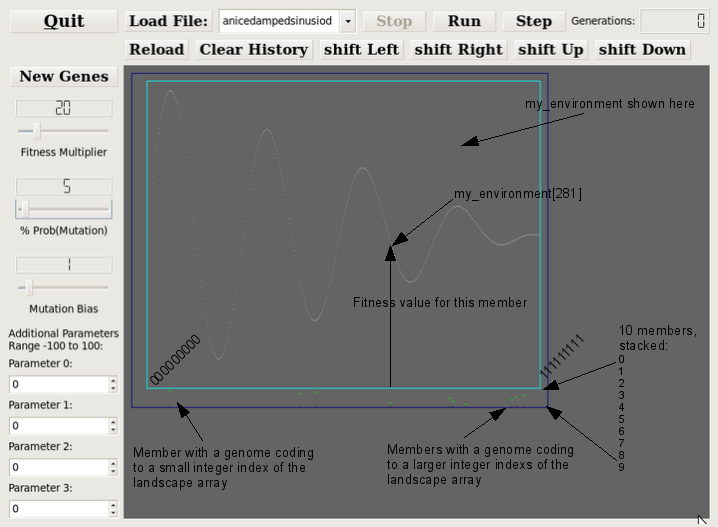### Initial Population

 GAs begin with a "population" of "members", where each of the members consist of a complete coding of the problem's parameters. In this example, the population is M members consisting of log2(N) randomly selected bits (which, as an integer, form the index into the array). These N bits are the "genome". The population of `gaga` is `my_community` which is `MEMBERS` length array of `GENOME_BITS` of integers (but only 0 and 1 are legitimate values for those ints). ```#define MEMBERS 10 #define GENOME_BITS 9 #define ENVIRONMENT_LENGTH 512 int my_community[MEMBERS][GENOME_BITS], int my_environment[ENVIRONMENT_LENGTH], ``` There are 10 `MEMBERS` and they are represented below as green bars. There are 9 `GENOME_BITS`, corresponding to an indexible landscape of 0:511. Green bars to the left represent those members with smallish indexes, those on the right represent members with indexes towards the high end.Clicking the "New Genes" button repopulates the 10 members with new bits (compare the position of the green bars in the image above with the image below).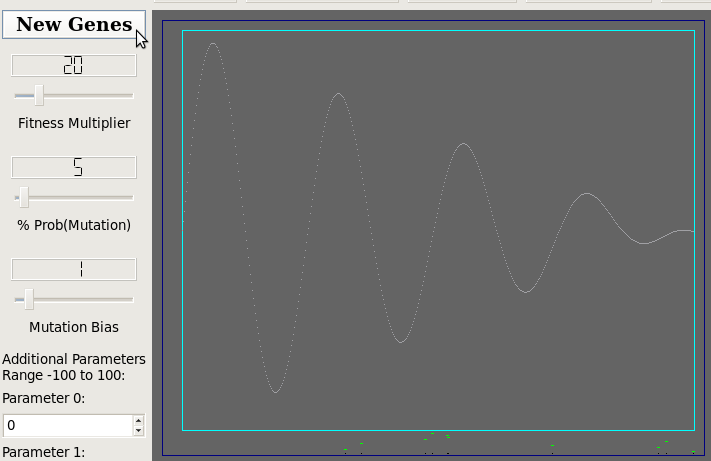### Fitness Function

 Whatever the method is for evaluating the expression for different values of the code (genome), that method is applied here. The particular method for this example is simply returning the value of the array using the genome of each member as an integer index. The returned value is the fitness. The complete "fitness function" could be more complex, such as applying a linear or exponential scaling of the returned value. It could include aspects the member's history such as a running average or the number of generations without changes to the genome. Here, we treat the `GENOME_BITS` as an integer index to the environment and at first consider the fitness to be just the return value. Then all member's fitness values are shifted down by the lowest fitness value. Then all are multiplied by that fitness multiplier. ```... my_mmbrsInt[membr] = genomeAsInteger(GENOME_BITS, my_community[membr]) ; fitness[membr] = my_environment[my_mmbrsInt[membr]] ; ... fitness[membr] = fitness[membr] - lowest ; fitness[membr] = fitness_multiplier * fitness[membr] ; ... ``` The screenshot fragment shows the control for passing the fitness multiplier to `gaga`.### Reproduction Function

 The M members have been graded by the fitness function. Some are more "fit" than others. These are the members we are most interested in keeping and will feature in the reproduction (or "breeding" or "mating") process. There are three primary steps to this reproduction as we plan to wipe out all members' genomes and replace them with genomes (new bits). The new members for the next iteration will be randomly selected from a pool of the old members, but the selection will be biased towards those most fit members. The steps are: 1. Create a "weighted roulette wheel", an array of values where each of the members is represented in proportion to thier fitness value. More fit members occupy more space in the array. This is done in a quite straight-forward way by populating an array with a number of member numbers, in proportion to thier fitness. This provides the bias towards the most fit. This is how the weighted wheel is implemented in `gaga`: ``` ww = (int*) (malloc(total_fitness*sizeof(int))) ; ... for (membr=0;membr

### Mutation Function

 Sometimes, things go wrong with the whole reproduction business. Occasionally one of the coded parameter values is copied incorrectly. At least we pretend it does. In this example all of those values are bits, so we "flip a bit" to mimic this loss of fidelity. Ok. So the code below is a bit rococo ... but it is always in flux. ``` int b_random_num ; for (membr=0;membr acc) && (g < GENOME_BITS)) { g++ ; acc += mutation_biases[g] ; } b_random_num = g ; } else { b_random_num = rand() % (GENOME_BITS) ; } // This "1 if 0, 0 if 1" code could be simpler but it is left // as a placeholder (I was considering using the remaining bits // of each int for statistics rather than just using an int // as only a 1 or 0. if (my_mmbrsNew[membr][b_random_num] == 0) { my_mmbrsNew[membr][b_random_num] = 1 ; } else { my_mmbrsNew[membr][b_random_num] = 0 ; } } } } ``` Here are screenshot fragments of the two controllers for mutation_probability and my_mutation_bias.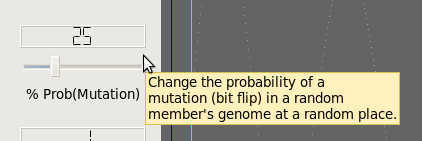### Termination Criteria

 Just like each of the M members in the initial population was evaluated, so too are the new "generation" of members evaluated. As generations go by the population diversity should shrink as the entire population converges towards representing the integer index of array returning the largest value. The decision of when to stop iterating and present a final population is what the "stopping" or "termination criteria" is about. Like other aspects of GAs, it is the subject of much research. Common conditions for stopping are: A solution is found that satisfies minimum criteria Fixed number of generations reached Allocated budget (computation time/money) reached The highest ranking solution's fitness is reaching or has reached a plateau such that successive iterations no longer produce better results Manual inspection Combinations of the above There is no actual stopping criteria built into `gaga`. It will run and modify the population's genome and return. Any termination decision must be made elsewhere. The collection of screenshot fragments below shows several successive clicks of the "Step" button. Each step resulted in a convergence of the 10 member's genomes towards the high point of the curve. The stopping criteria was simple: stop clicking the "Step" button when I've accumulated an illustrative set of screenshots.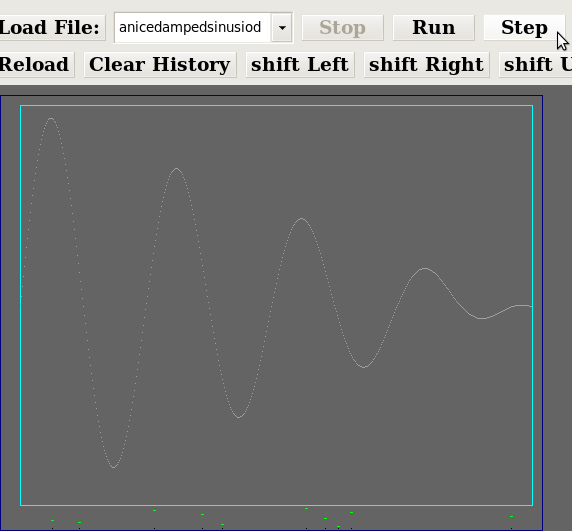Step 1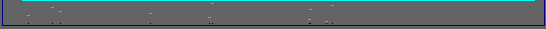Step 2Step 3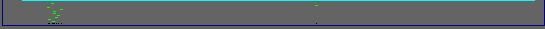Step 4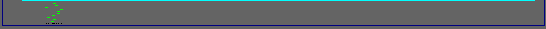Step 5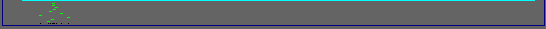Step 6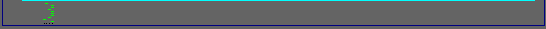Step 15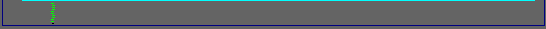Step 17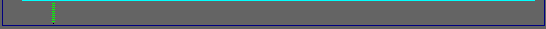Step 18

That ends the tour of the GA `gaga` and the graphical interface for a nine-bit instance of `gaga`.

### Just the genetic algorithm itself

Here is a gzipped tar file of the genetic algorithm source gaga.tar.gz.

### The QtCreator project

Here is a gzipped tar file with the QtCreator project (Qt Creator 2.0.1, Based on Qt 4.7.0 32 bit). It includes the gaga code. GAGA9B.tar.gz.

## Future work

This demo is obviously a toy. But it is one which I have spent a great deal of time observing and changing. When I return to it next I will make the following changes:
• Recode the genome.
(There are issues with a simple binary encoding of the genome, most notably the Hamming wall. A Gray code may be in order.)
• Add a "Set Population" feature to allow user specified inital populations.
• Add a record an playback feature to record everything, including all the random numbers generated.
• Build a set of run-time selectable reproduction options.
• Include selectable and parametric stopping functions.

## Presentation

Feel free to contact me with questions or comments. I have also put together a show-and-tell presentation. You will need to contact me for the tell part, but it is shown here (OpenOffice Presentation): gaga.odp.